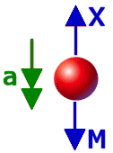Go to contentNewton's law states that F=ma.

F stands for the resultant force, in Newtons, acting on a particle,
m is the mass in kilograms of the particle
a is the acceleration in m/s² produced.

Newton's law can be applied in force diagrams to find various forces acting on a particle.

## Summary/BackgroundSir Isaac Newton (4 January 1643 - 31 March 1727) was the greatest English mathematician of his generation. He laid the foundation for differential and integral calculus. His work on optics and gravitation make him one of the greatest scientists the world has known.
Newton's laws of motion are three physical laws which provide relationships between the forces acting on a body and the motion of the body, first compiled by Sir Isaac Newton. Newton's laws were first published together in his work Philosophiae Naturalis Principia Mathematica (1687). The Principia is recognised as the greatest scientific book ever written. Newton analysed the motion of bodies in resisting and non-resisting media under the action of centripetal forces. The results were applied to orbiting bodies, projectiles, pendulums, and free-fall near the Earth. He further demonstrated that the planets were attracted toward the Sun by a force varying as the inverse square of the distance and generalised that all heavenly bodies mutually attract one another.

The laws form the basis for classical mechanics.
1. Every object in a state of uniform motion tends to remain in that state of motion unless an external force is applied to it.
2. The relationship between an object's mass m, its acceleration a, and the applied force F is F = ma. Acceleration and force are vectors (as indicated by their symbols being displayed in slant bold font); in this law the direction of the force vector is the same as the direction of the acceleration vector.
3. For every action there is an equal and opposite reaction.You can get a better display of the maths by downloading special TeX fonts from jsMath. In the meantime, we will do the best we can with the fonts you have, but it may not be pretty and some equations may not be rendered correctly.

## Glossary

### acceleration

the rate of change of velocity with time. It is a vector quantity with magnitude and direction.

### body

an object with both mass and size that cannot be taken to be a particle

### calculus

the study of change; a major branch of mathematics that includes the study of limits, derivatives, rates of change, gradient, integrals, area, summation, and infinite series. Historically, it has been referred to as "the calculus of infinitesimals", or "infinitesimal calculus".
There are widespread applications in science, economics, and engineering.

### centripetal

of a force directed towards the centre in circular motion

### force

that which causes a body to accelerate or change in momentum

### integral

the anti-derivative

### mass

a measure of the quantity of matter in an object

### newton

the unit of force

### particle

an object with negligible size and internal structure, which can be represented by a point; the fundamental assumption in mechanics.

### union

The union of two sets A and B is the set containing all the elements of A and B.

### vector

A mathematical object with magnitude and direction.

### work

Equal to F x s, where F is the force in Newtons and s is the distance travelled and is measured in Joules.

Full Glossary List

## This question appears in the following syllabi:

SyllabusModuleSectionTopicExam Year
AQA A-Level (UK - Pre-2017)M1DynamicsNewton's Laws-
AQA AS Maths 2017MechanicsForces and Newton's LawsNewton's Laws-
AQA AS/A2 Maths 2017MechanicsForces and Newton's LawsNewton's Laws-
CCEA A-Level (NI)M1DynamicsNewton's Laws-
CIE A-Level (UK)M1DynamicsNewton's Laws-
Edexcel A-Level (UK - Pre-2017)M1DynamicsNewton's Laws-
Edexcel AS Maths 2017MechanicsForces and Newton's LawsNewton's Laws-
Edexcel AS/A2 Maths 2017MechanicsForces and Newton's LawsNewton's Laws-
OCR A-Level (UK - Pre-2017)M1DynamicsNewton's Laws-
OCR AS Maths 2017MechanicsForces and Newton's LawsNewton's Laws-
OCR MEI AS Maths 2017MechanicsForces and Newton's LawsNewton's Laws-
OCR-MEI A-Level (UK - Pre-2017)M1DynamicsNewton's Laws-
Pre-U A-Level (UK)MechDynamicsNewton's Laws-
Universal (all site questions)DDynamicsNewton's Laws-
WJEC A-Level (Wales)M1DynamicsNewton's Laws-